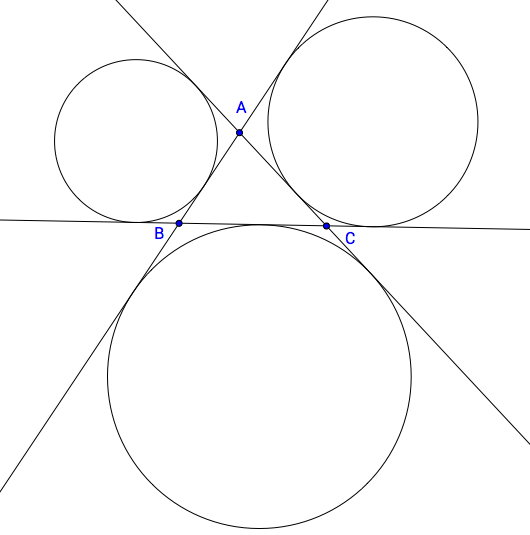# Three Lines, Three Circles

Geometry Level 5Lines $l, m, n$ in the same plane are such that $l \cap m = A,$ $m \cap n = B,$ $l \cap n = C,$ and $A \neq B \neq C.$ The three circles that are tangent to $l, m,$ and $n$ but not in the interior of $ABC$ have radii $3, 4,$ and $5.$ If $AB + BC + CA = p\sqrt{q},$ for positive integers $p$ and $q$ such that $q$ is not divisible by the square of any prime, find $p+q.$

Notes:

Assume that such a configuration described in the problem is possible.

$a \cap b$ means the intersection of lines $a$ and $b.$

See the next problem here.

×